Back to Kemp Acoustics HomeNext: Multimodal equations in a Up: Multimodal propagation in acoustic Previous: Plane waves in a   Contents

# Plane waves at a change in cross-section

A wave experiences partial reflection and partial transmission at a change in impedance. Such a change occurs at a change in cross-sectional area since the characteristic impedance depends on
. Consider the discontinuous join between two cylinders shown in figure 2.2.
We label as plane 1 the cross-section with area
immediately to the left of the discontinuity. Plane 2 is defined to be the cross-section with area
immediately to the right. The pressure and volume velocity on either side of a change of cross-section are equal, meaning thatand
in the current notation. It follows that the acoustic impedance is the same on each side of the discontinuity (ie.
).

It should not seem strange that the acoustic impedance is unaltered by the change in cross-section because the characteristic impedance
has changed and the acoustic impedance has therefore altered relative to the characteristic impedance. We may use equation (2.13) to work out the impedance at plane 0 and the effect of the new cross-section will then have an effect on the resultingvalue.

To further illustrate the point, we must deal with the forward and backward going waves in each cylinder.
and
are the complex pressure amplitudes for the waves in the cylinder to the left of discontinuity, meaning the pressure there from equation (2.2) is
(2.14)
and
are the forward and backward going complex pressure amplitudes in the cylinder on the right of the discontinuity giving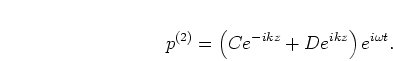(2.15)

Usingat the discontinuity (noting that planes 1 and 2 may be taken to be at the same
coordinate, just on opposite sides of the infinitely sharp discontinuity) gives
(2.16)

From equation (2.7) the we volume velocity in plane 1 is
(2.17)

and the volume velocity in plane 2 is
(2.18)

Using thecondition leads to
(2.19)

While the sum of the forward and backward waves is the same on either side of the discontinuity, the difference depends on the ratios of the cross-sections, hence the waves experience reflection and transmission coefficients.

In particular, consider if our pipes in figure 2.2 are infinite in length. A pressure wave is incident on the discontinuity from
with amplitude. It is partially reflected with amplitudeback down the tube toand partially transmitted towith amplitude.
since there is no backward going wave in. We can solve (2.16) and (2.19) by eliminatingto get the reflection coefficient:
(2.20)

A decrease in area means a positive reflection coefficient and an increase in area means a negative reflection coefficient while there is no reflection when
. The transmission coefficient is obtained by eliminatingin the same equations:
(2.21)

To summarise, impedance is not effected by changes in cross-section and equation (2.13) describes how impedance changes when projected along a pipe of given cross-section. Now we have the equations necessary to work out the impedance at one end of a instrument of known internal profile provided the impedance at the other end is known. The impedance at the open end will depend on the geometry of the opening and is treated in detail in chapter 3. Projecting the impedance down to the input (mouthpiece) end gives us the input impedance, the amount of pressure produced in the mouthpiece by a unit volume velocity source. Now we have derived a method of input impedance calculation in the plane wave approximation, we will go on to do the same for multimodal propagation.

Back to Kemp Acoustics HomeNext: Multimodal equations in a Up: Multimodal propagation in acoustic Previous: Plane waves in a   Contents
Jonathan Kemp 2003-03-24